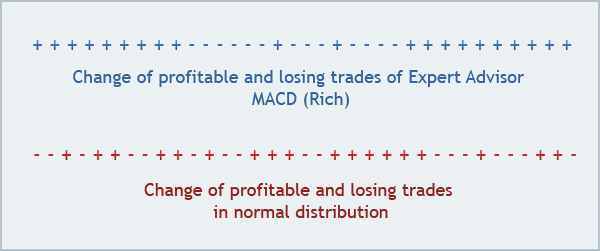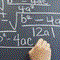Z-Score11613

Hi,

Regarding the formula of the Z Score (found @ https://www.mql5.com/en/articles/1492),

Z=(N*(R-0.5)-P)/((P*(P-N))/(N-1))^(1/2)

where:
N - total amount of trades in a series;
R - total amount of series of profitable and losing trades;
P = 2*W*L;
W - total amount of profitable trades in the series;
L - total amount of losing trades in the series.

For such a series (the one at the top) :We'll use :

N = 34
R = 5 (2 + / 3 -)
P = 2*W*L;
W - total amount of profitable trades in the series; <---- for W & L, is it the total amount of win deals & loss deals, in a way that : W+L = 34 ?
L - total amount of losing trades in the series.
Correct ?Mathematics in Trading: How to Estimate Trade Results
• 2007.08.15
• MetaQuotes Software Corp.
• www.mql5.com
We all are aware of that "No profit obtained in the past will guarantee any success in future". However, it is still very actual to be able to estimate trading systems. This article deals with some simple and convenient methods that will help to estimate trade results.